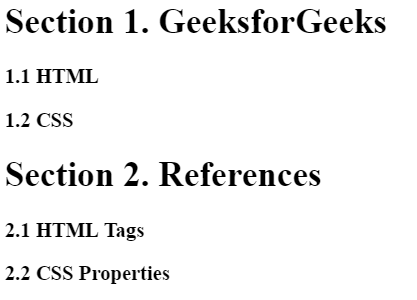Related Articles
CSS | counter-reset Property
• Last Updated : 06 Feb, 2019

The counter-reset property in CSS is used to create or reset the CSS counter for elements. This property works together with counter-increment property and the content property.

Syntax:

`counter-reset = none|name number|initial|inherit;`

Property Values:

• none: It is the default value. This value does not reset the counter.
• name number: The value to reset the counter on each occurrence of the element. The default value is 0 if not specified.
• initial: It sets the counter-reset property to its default value.
• inherit: The element inherits the property from its parent element.

Example 1: This example use counter-reset property to create section.

 ` ` `<``html``> ` `     `  `<``head``> ` `     `  `    ``` `    ``<``style``> ` `         `  `        ``/* set chapter counter to 0*/ ` `        ``body { ` `            ``counter-reset: chapter;      ` `        ``} ` `        ``.chapter:before { ` `            ``content: counter(chapter) ". "; ` `            ``display: inline; ` `        ``} ` `        ``.chapter { ` `             `  `            ``/* Increment the chapter counter by 1, ` `            ``same as counter-increment: chapter 1; */ ` `            ``counter-increment: chapter; ` `             `  `            ``/* set section counter to 0 */ ` `            ``counter-reset: section;      ` `            ``font-size: 20px; ` `            ``font-weight: bold; ` `        ``} ` `    `` ` ` ` ` `  `<``body``> ` `    ``<``h1``>GeeksforGeeks ` `     `  `    ``<``div` `class` `= ``"chapter"``>HTML ` `    ``<``div` `class` `= ``"chapter"``>CSS ` `    ``<``div` `class` `= ``"chapter"``>PHP ` `                     ` ` `  ` `

Output:Example 2: This example use counter-reset property to create section and subsection.

 ` ` `<``html``> ` `     `  `<``head``> ` `     `  `    ``` `    ``<``style``> ` `        ``body { ` `            ``counter-reset: section; ` `        ``} ` `        ``h1 { ` `            ``counter-reset: category; ` `        ``} ` `        ``h1:before { ` `            ``counter-increment: section; ` `            ``content: "Section " counter(section) ". "; ` `        ``} ` `        ``h3:before { ` `            ``counter-increment: category; ` `            ``content: counter(section) "." counter(category) " "; ` `        ``} ` `    `` ` ` ` ` `  `<``body``> ` `    ``<``h1``>GeeksforGeeks ` `     `  `    ``<``h3``>HTML ` `    ``<``h3``>CSS ` `     `  `    ``<``h1``>References ` `     `  `    ``<``h3``>HTML Tags ` `    ``<``h3``>CSS Properties ` ` ` ` `  `                     `

Output:Supported Browsers: The browser supported by counter-reset property are listed below: